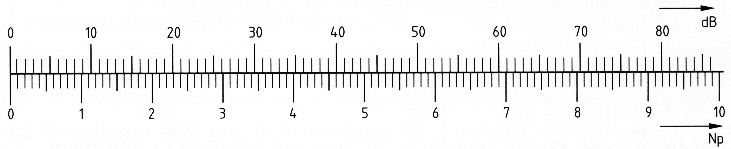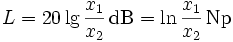Deutsche VersionConversion ratios of gain and loss

Level with the pseudo units:
neper (Np), decibel (dB), and bel

Enter the known pseudo unit at the appropriate line.
The other boxes will show the conversions.
You don't need a calculate bar.

 Bel B Decibel dB Neper Np ← reset

Notice: Do not re-enter the exact number of an answer.The conversion scale of the pseudo units decibel (dB) and neper (Np)

 Neper is a unit used to express ratios, such as gain, loss, and relative values. Note 1: The neper is analogous to the decibel, except that the Naperian base 2.718281828 is used in computing the ratio in nepers. Note 2: The value in nepers is given by Np = ln (x1 / x2), where x1 and x2 are the values of interest, and ln is the natural logarithm, i.e., logarithm to the base e. Note 3: One neper Np ≡ 20 / (ln10) = 8.685889638 dB. Note 4: The neper is often used to express voltage and current ratios, whereas the decibel is also used to express power ratios. Note 5: Like the decibel dB, the Np is a dimensionless unit. Note 6: The ITU recognizes both units.

 The other likely candidate for the base of the log is e = 2.718281828, which has nice properties in equations, etc., and just seems to fall out of all sorts of natural phenomena - unlike 10, which is popular because we have 10 fingers. Using e as your base leads to a less common unit called the neper (Np). Neper is the Latin form of Napier, the guy who popularized the use of logs for computation (viz. Napier's bones). Nepers are used with voltage (or magnitude) ratios, so watch out when converting back and forth between nepers and dB. Decibel and neper have a fixed linear ratio to each other.   1 Np ≡ 20 / ln(10) = 8.685889638 dB 1 dB ≡ ln(10) / 20 Np = 0.115129255 Np       1 Np = (20 lg e) dB ≈ 8.6859 dB               1 dB = (0.05 ln 10) Np ≈ 0.11513 Np                   Conversion from dB to Np: Np value ≡ dB value × ln (10) / 20 = dB value × 0.115129255   Conversion from Np to dB: dB value ≡ Np value × 20 / ln (10) = Np value × 8.685889638   The voltage level isx2 is the reference value. John Napier of Merchiston (1550 - 4 April 1617) - also signed as Neper, Nepair - named Marvellous Merchiston, was a Scottish landowner known as a mathematician, physicist, astronomer and astrologer.

Conversion: bel, decibel, centibel, and millibel

 The Bel is no physical size, but means only that it is the value of the logarithm of a ratio. Sound pressure level (SPL) is the ratio of sound pressure in comparison to a reference sound pressure, the threshold of hearing (0.00002 Pa = 20 µPa).